# 基于ArkUI开发框架，图片马赛克处理的实现

HarmonyOS开发者

1收藏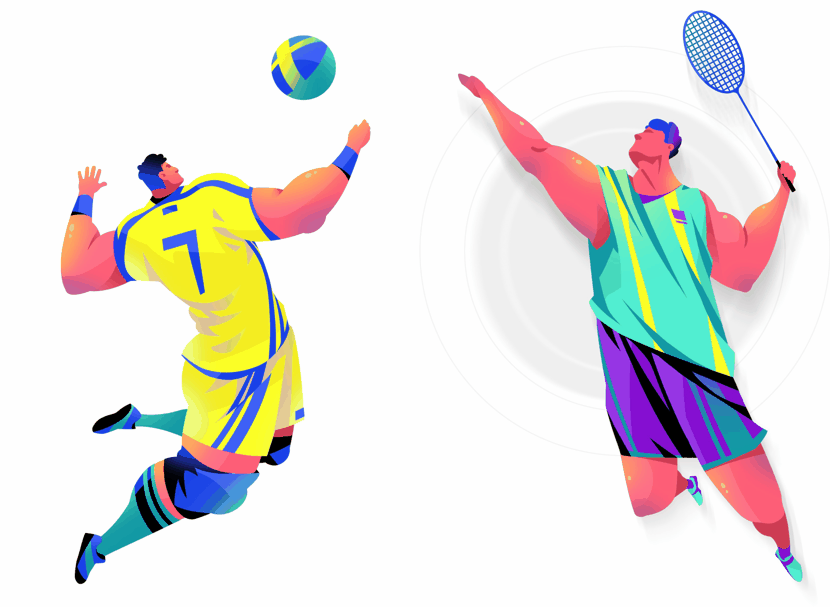1. 像素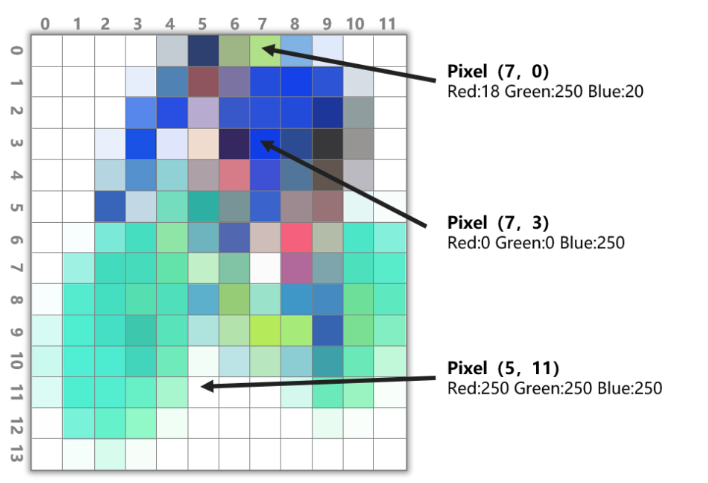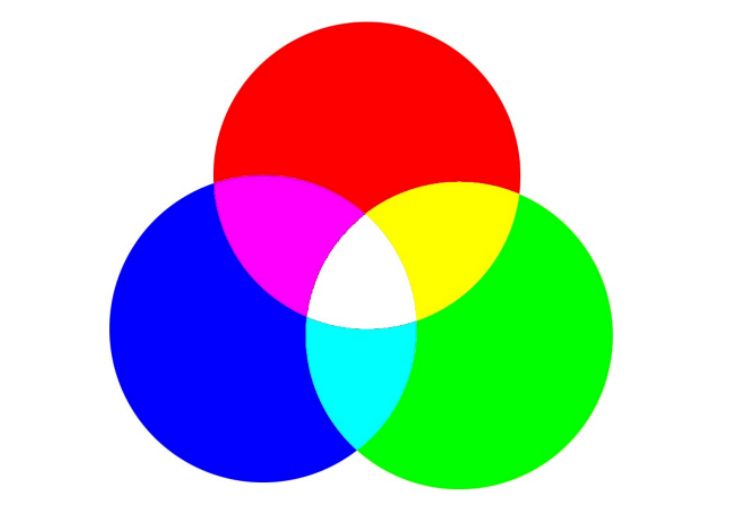2. 分辨率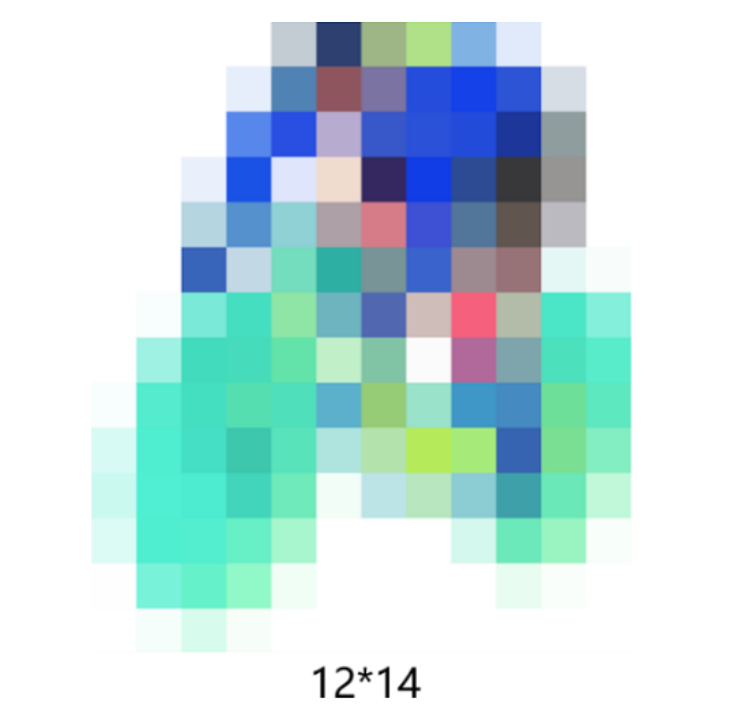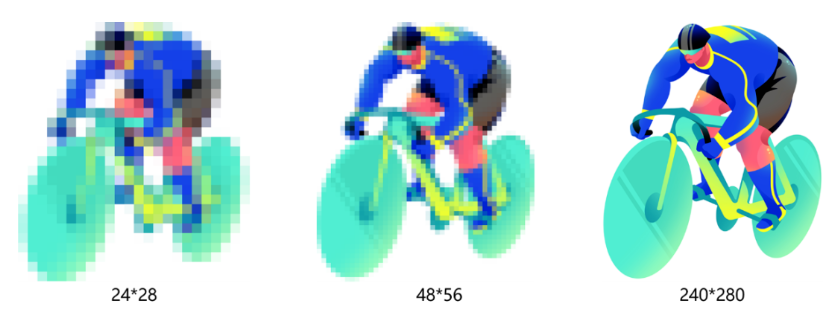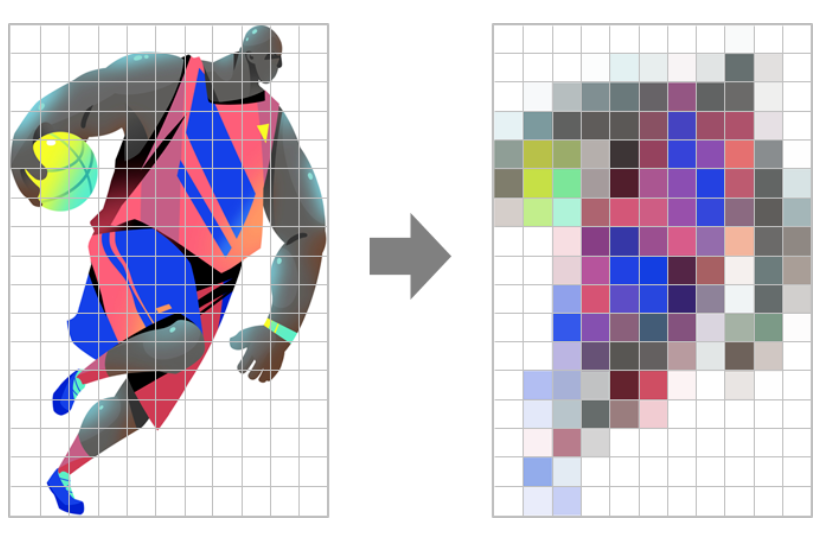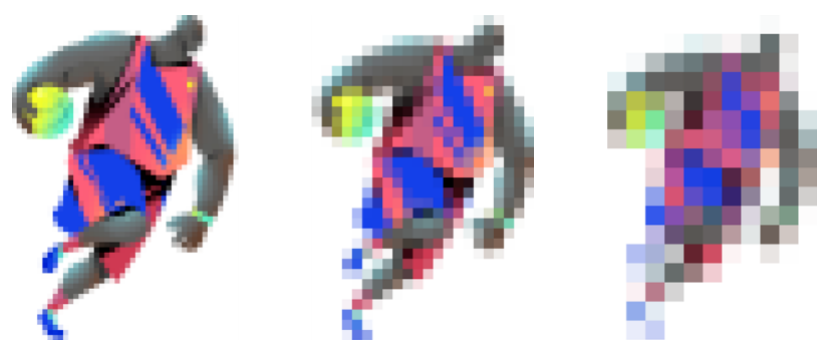1. 首先我们需获取ArkUI开发框架的image能力，该能力提供了图片开发的基本接口。

``import image from "@ohos.multimedia.image"``

``````let bufferData = new ArrayBuffer(bitmap.getPixelBytesNumber());

```​for (let index = 0; index < dataArray.length; index += 4) { const r = dataArray[index]; const g = dataArray[index+1]; const b = dataArray[index+2]; const a = dataArray[index+3]; }​```

3. 根据自定义的单个小方格的Width和Height，将整个图片分成若干小方格。

``````//横排的正方形个数

var x_index = Math.floor(targetWidth / realPixel_W);

//纵排的正方形个数

var y_index = Math.floor(targetHeight / realPixel_H);``````

4. 获取每个小方格左上角的最大坐标及右下角的最小坐标，以确定小方格的区域。并根据每个小方格内的所有像素点数据统一该区域的像素，统一方式可以是取该区域内像素点的平均值，或者随机选取一个像素。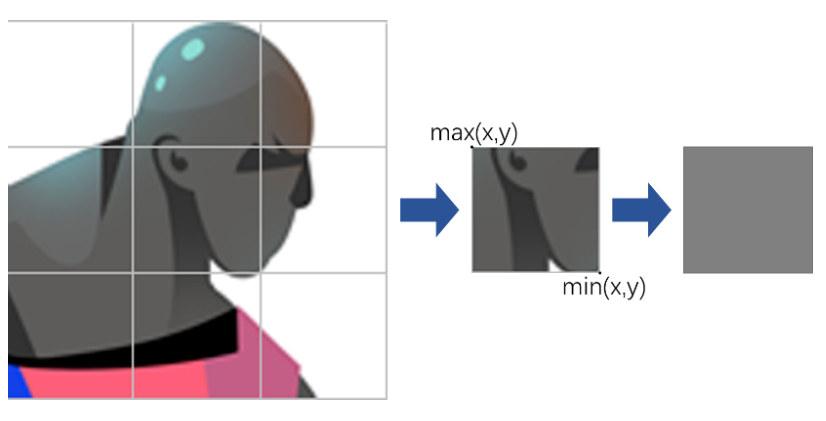``````for (let ch = 0; ch < y_index; ch++) {

for (let cw = 0; cw < x_index; cw++) {

let max_x = (cw + 1) * realPixel_W > targetWidth ? targetWidth : (cw + 1) * realPixel_W;

let max_y = (ch + 1) * realPixel_H > targetHeight ? targetHeight : (ch + 1) * realPixel_H;

let min_x = cw * realPixel_W;

let min_y = ch * realPixel_H;

//取左上角的像素值

let center_p = inPixels[min_y+1][min_x+1];

//设置该正方形里的像素统一

for (let zh = min_y; zh < max_y; zh++) {

for (let zw = min_x; zw < max_x; zw++) {

inPixels[zh][zw] = center_p;

}

}

}

}``````

5. 通过writeBufferToPixels接口，将统一的像素点数据缓存到ArrayBuffer中，并写入PixelMap，由此得到整张马赛克处理的图片。

``writeBufferToPixels(src: ArrayBuffer): Promise<void>``

1. 给图片添加Touch事件，获取手指的运动轨迹。参考代码如下：

``````Image(this._mCropPixelMap.pixelMap)

.width(300)

.height(300)

.margin(10)

.objectFit(ImageFit.Contain)

.onTouch(event => {

let array: TouchObject[] = event.changedTouches;

for (let i = 0;i < array.length; i++) {

//触摸的x y坐标

let centX = array[i].x;

let centY = array[i].y;

}

});``````

2. 根据运动轨迹，以触摸点的坐标（x，y）为中心，根据自定义小方格的大小，动态确认马赛克区域的位置。参考代码如下：

``````//获取到左上角的坐标

let offMinX = Math.floor(centerX - pixel / 2);

let offMinY = Math.floor(centerY - pixel / 2);

//右下角的坐标

let offMaxX = Math.floor(centerX + pixel / 2);

let offMaxY = Math.floor(centerY + pixel / 2);

offMinX = offMinX < 0 ? 0 : offMinX;

offMinY = offMinY < 0 ? 0 : offMinY;

offMaxX = offMaxX > targetWidth ? targetWidth : offMaxX;

offMaxY = offMaxY > targetHeight ? targetHeight : offMaxY;``````

3. 统一马赛克区域的所有的像素点值。

``````//取左上角的像素值

let center_p = PixelExampleUtils.inPixels[offMaxY+1][offMaxX+1];

//设置该正方形里的像素统一

for (let zh = offMinY; zh < offMaxY; zh++) {

for (let zw = offMinX; zw < offMaxX; zw++) {

PixelExampleUtils.inPixels[zh][zw] = center_p;

changeArray[zh][zw] = center_p;

}

}``````

4. 最后将更改的像素点写入图片中，即可得到手指滑动轨迹的马赛克图片。1